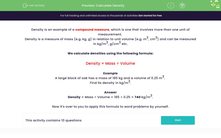# Calculate Density

In this worksheet, students will be asked to work out densities given the mass and volume of objects.Key stage:  KS 3

Curriculum topic:   Geometry and Measures

Curriculum subtopic:   Derive and Apply Formulae

Difficulty level:#### Worksheet Overview

Density is an example of a compound measure, which is one that involves more than one unit of measurement.

Density is a measure of mass (e.g. kg, g) in relation to unit volume (e.g. m3, cm3) and can be measured in kg/m3, g/cm3 etc.

We calculate densities using the following formula:

Density = Mass ÷ Volume

Example

A large block of oak has a mass of 185 kg and a volume of 0.25 m3

Find its density in kg/m3.

Density = Mass ÷ Volume = 185 ÷ 0.25 = 740 kg/m3.

Now it's over to you to apply this formula to word problems by yourself.### What is EdPlace?

We're your National Curriculum aligned online education content provider helping each child succeed in English, maths and science from year 1 to GCSE. With an EdPlace account you’ll be able to track and measure progress, helping each child achieve their best. We build confidence and attainment by personalising each child’s learning at a level that suits them.

Get started